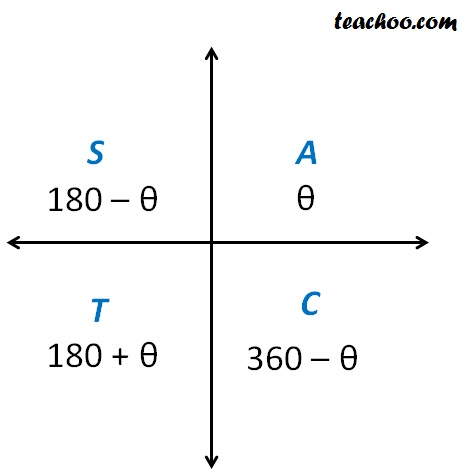Finding Principal solutions

Chapter 3 Class 11 Trigonometric Functions
Concept wise

### Principal solution for sin x = ½

sin x = ½

Here sin is positive,We know that

sin is positive in 1st and 2nd quadrant

Value in 1st Quadrant = 30°

Value in 2nd Quadrant = 180° – 30° = 150°

So, Principal solutions are

x = 30° = 30° × π/180 = π/6

x = 150° = 150° × π/180 = 5π/6

Thus, Principal solutions are

π/6 & 5π/6

### Principal solution for cos x = –1/√2

cos x = –1/√2

Here cos is negative,We know that

cos is negative in 2nd and 3rd quadrant

Here, θ = 45°

Value in 2nd Quadrant = 180° – 45° = 135°

Value in 3rd Quadrant = 180° + 45° = 225°

So, Principal solutions are

x = 135° = 135° × π/180 = 3π/4

x = 225° = 225° × π/180 = 5π/4

Thus, Principal solutions are

3π/4 & 5π/4

### Principal solution for tan x = –1

tan x = –1

Here tan is negative,We know that

tan is negative in 2nd and 4th quadrant

Here, θ = 45°

Value in 2nd Quadrant = 180° – 45° = 135°

Value in 4th Quadrant = 360° – 45° = 315°

So, Principal solutions are

x = 135° = 135° × π/180 = 3π/4

x = 315° = 315° × π/180 = 7π/4

Thus, Principal solutions are

3π/4 & 7π/4

Learn in your speed, with individual attention - Teachoo Maths 1-on-1 Class

### Transcript

Principal solution for sin x = sin x = Here sin is positive, We know that sin is positive in 1st and 2nd quadrant Value in 1st Quadrant = 30 Value in 2nd Quadrant = 180 30 = 150 So, Principal solutions are x = 30 = 30 /180 = /6 x = 150 = 150 /180 = 5 /6 Thus, Principal solutions are /6 & 5 /6 Principal solution for cos x = 1/ 2 cos x = 1/ 2 Here cos is negative, We know that cos is negative in 2nd and 3rd quadrant Here, = 45 Value in 2nd Quadrant = 180 45 = 135 Value in 3rd Quadrant = 180 + 45 = 225 So, Principal solutions are x = 135 = 135 /180 = 3 /4 x = 225 = 225 /180 = 5 /4 Thus, Principal solutions are 3 /4 & 5 /4 Principal solution for tan x = 1 tan x = 1 Here tan is negative, We know that tan is negative in 2nd and 4th quadrant Here, = 45 Value in 2nd Quadrant = 180 45 = 135 Value in 4th Quadrant = 360 45 = 315 So, Principal solutions are x = 135 = 135 /180 = 3 /4 x = 315 = 315 /180 = 7 /4 Thus, Principal solutions are 3 /4 & 7 /4